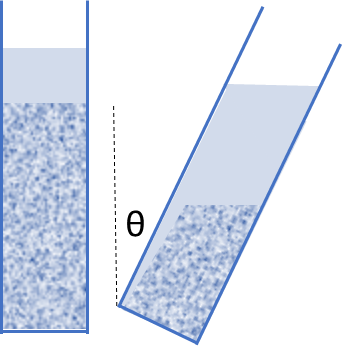## Stokes Sedimentation Calculator + Boycott Effect

### Quick Start

This App uses Stokes’ law to estimate the rate at which a dispersion will settle. It will help you to predict how settling will depend on properties of your formulation such as

• Viscosity, shown as η
• Density of dispersed and continuous phases, shown as ρ

You will need to know or be able to estimate these properties plus a few more such as volume fraction φ.

The amazing Boycott effect which greatly increases the settling rate in inclined tubes was added in Jan 2023 when foam scientist Dr Paul Stevenson told me of its existence, and how it works upside-down for foams.

### Stokes Sedimentation

Particle r nm
ρp g/cc
ρl g/cc
Viscosity η cP
Aspect Ratio
Settling height h mm
Width b mm
Angle θ
φ
RPM
v m/s
Time
grel
Gr: Gravity v kT
Too small to settle?

When you have particles of radius r of density ρp in a liquid of viscosity η and density ρl and a gravitational force g then the particles will fall at a velocity v and cover a distance h in time t. The formula is the Stokes equation:

v=2.18(ρ_p-ρ_1)g_(rel)r^2/η

t=h/v

g_(rel)=1+0.001118 r_(cent) RPM^2

The normal formula uses g for gravity but if the particle is spinning at a given RPM in a centrifuge of radius rcent then the effective force is larger, grel (relative to g) and the settling is faster. The 2.18 in the formula is the familiar 2/9 from the Stokes equation multiplied by g=9.81. The significance of φ is explained below as are Width and θ.

If your particle isn't spherical it has an aspect ratio, AR, (long dimesion/short dimension greater than 1, and the Stokes equivalent radius is reduced. If AR21 = Sqrt(AR²-1) then the effective radius, reff is given by1:

r_(eff)=r.sqrt((arctan(AR21))/(AR21))

If ρp is less than ρl as in a typical oil in water emulsion then the "settling" velocity is negative and t is the time taken for the emulsion particle to rise by h.

So far, we have assumed that the particles don't interfere with themselves. A simple, but effective approximation from Richardson and Zaki tells us that the velocity is reduced by a factor of (1-φ)5.65 where φ is the volume fraction. (As pointed out by Dr Paul Stevenson, the power is normally quoted as 4.65 but that's the Lagrangian view, for our Eulerian view it's 5.65). Clearly this means that as particles start to settle and get locally more concentrated, the rate will reduce. This app is just to give a general idea of the effects in the early stages of settling.

There is one more factor. The argument goes that if the particle is very small then thermal motion (kT) is enough to keep it moving randomly so it will not settle. This is controlled by a Gravity Number, Gr given by:

Gr = (0.384.2πr^4Δρg)/(3kT)

The 0.384 comes from some assumptions about relative particle sizes and is a maximum value for a size ratio of 1.66:1. For simplicity, T is assumed to be 298K. The "Too small to settle" box goes to "Yes" when Gr < ~1 For the case of 1g, most nanoparticles will not settle easily. But as you spin the rotor, it is harder for thermal motions to resist the higher g.

It happens that this argument is bogus, but in practice smaller particles with the normal vibrations, convection currents etc. can resist settling for some considerable time. To see why it is bogus, explore the Gravitational Sedimentation app.

### The Boycott effectIn the 1920's, Boycott noticed that red blood cells settled much faster in a tilted tube than in a vertical one. The effect is real. During the settling, a thin clear layer appears between the particles and the upper sloped wall. There are various explanations for the effect. One says that the particles have a smaller distance to fall till they reach the wall and can then slide down the wall. I prefer the equivalent view that the clear layer is a back channel for solvent displaced by the falling particles to escape - something not possible in a vertical tube.

There are many subtleties and edge cases, but the general PNK theory from the 1920's/30's is good enough for us and is fortunately simple. Where H is the height of the particle column, b is the width of the tube (well, the theory assumes parallel plates) and the tilt angle is θ, defined as 0 for a vertical tube, if we start with the velocity v calculated above then the new Boycott velocity, VB is given by:

v_B = v(1+H/bsinθ)

Clearly the simple theory breaks down if H/b is very large because flow rates become large enough for other effects (Reynolds number and Grashof number) to become significant.

### Notes and limitations

Although measuring densities and viscosities is not too hard, you are unlikely to have a single, known radius. In any case, as particles start to settle, they get slowed down either according to Richardson and Zaki or by other effects such as flocculation according to Buscall-White theory. So the point of the app is to give a feel for the sort of formulation options available to you, such as changing the viscosity and density of the liquid.

1B. R. Jennings and K. Parslow, Particle Size Measurement: The Equivalent Spherical Diameter, Proc. Royal Soc. London. Series A, Mathematical and Physical Sciences, 419, No. 1856. 1988, 137-149